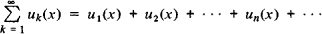# Domain of Convergence

The following article is from The Great Soviet Encyclopedia (1979). It might be outdated or ideologically biased.

## Domain of Convergence

the set of values of a variable x for which a series of functionsconverges. The domain of convergence has a very simple form for power series. If a power series is considered for real values of the independent variable, then its domain of convergence is a single point, an interval (seeINTERVAL OF CONVERGENCE OF A POWER SERIES), which may contain one or both end points, or the entires-axis. If, however, complex values of the independent variable are also considered, then the domain of convergence of a power series is a single point, the interior of some circle (the circle of convergence), the interior of a circle and some points on the circumference, or the entire complex plane. Other types of series may have more complicated domains of convergence. For example, for a series of Legendre polynomials in the complex domain, the domain of convergence is the interior of an ellipse with foci at points — 1 and +1.

The domain of convergence is also defined for other processes. For example, the domain of convergence of an improper integral dependent on a parameter is understood to be the set of values of the parameter for which the given improper integral converges.

The Great Soviet Encyclopedia, 3rd Edition (1970-1979). © 2010 The Gale Group, Inc. All rights reserved.
References in periodicals archive ?
However, domain of convergence of equation (2) for the coefficients {[a.sub.k]} we will also call the Mandelbrot set.
It is necessary to determine the domain of convergence of expression (2) with respect to the variable [X.sub.n] and its change over time; and to compare these results with those obtained for the Mandelbrot set.
Therefore, Laplace-Pade resummation method  is used in literature to enlarge the domain of convergence of solutions or inclusive to find exact solutions.
In fact, the Laplace-Pade resummation method enlarges the domain of convergence of the truncated power series and often leads to an accurate approximation or the exact solution.
Next, in order to enlarge the domain of convergence of the truncated power series, a post-treatment combining Laplace transform and Pade approximant is applied.
This inequality is valid in the domain of convergence of the series it involves, that is, for y < [y.sub.T+1].
The domain of convergence of the series is given by [absolute value of s] < [rho].
If 0 < [rho] < 1, then the domain of convergence is the interior of D.
By computing [MATHEMATICAL EXPRESSION NOT REPRODUCIBLE IN ASCII] we see that (0, 2c) is included in the domain of convergence and that there is no [L.sup.p] boundedness for z [greater than or equal to] 2c.
It is possible to show a weaker form of Theorem 1 only in the [L.sup.2] domain of convergence without embedding as in (CDJH01).

Site: Follow: Share:
Open / Close